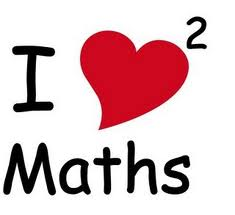# Basic mathematics  adding subtracting multiplication and division

## What will I learn from the English lesson on mathematics?

You will learn the English vocabulary for basic mathematics. The lesson shows examples in numbers and how to write the numbers in words (numeral).

### Vocabulary used for maths

 addition to add + subtraction to subtract - multiplication to multiply x division to divide : /

2 + 2 = 4                  two and two is/ are/ comes up to four

625 + 542 = 1167   six hundred and twenty-five plus five hundred and forty-two is/ equals one thousand, one hundred and sixty-seven

### Subtraction

6 – 4 = 2                   six take away four leaves/ is two

267 – 194 = 73        two hundred and sixty-seven minus one hundred and ninety-four equals seventy- three

### Multiplication

4 x 6 = 24                 four times six is/ makes twenty-four

42 x 63 = 2646        forty-two multiplied by sixty-three equals two thousand, six hundred and forty-six

### Division

24 : 6 =  4                 twenty-four divided by six equals four

## Lessons that are relatedTo view any of the lessons below click on link.

## Print the lesson

To print the lesson on Basic mathematics adding subtracting multiplication and division right click on a white space and choose print. You can click on the printer icon just below and to the right of the contact us menu button at the top of the page or copy and paste the part of the exercise you want onto a word document and then print onto some paper.

## Dictionary and how to use dictionaries

Click on the following link for the Online English dictionary - English lesson